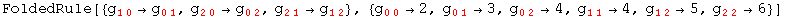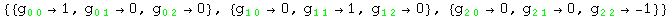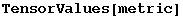7.7. Components of the metric, MetricInBasis

MetricInBasis        Values for the components of the metric

Components of the metric

It is very common in General Relativity to start working from a known line element. Inded, the typical approach is to start from the components of a metric in a coordinated basis, which determine both the metric and the coordinate system unambiguously. xCoba` provides a specialised function to define the values for the components of a metric, which can be used on its own or as an option for DefBasis.

In:=In:=Out=In:=In:=Out=In:=Out=An example with a diagonal metric

In:=In:=Out=And know with an orthonormal one

In:=In:=Out=In:=Out=In:=Out=Created by Mathematica  (May 16, 2008)